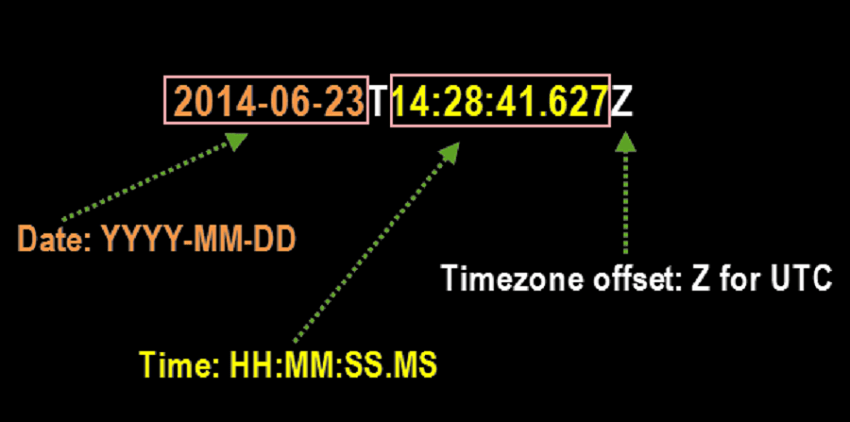# Formatting of ISO8601Date.java class in Open Event Android App

ISO8601Date.java is an util class in Open Event Android App which comprises of all the required date manipulation functionalities required by the application to parse the dates from the api given in ISO8601 format. Previous implementation of the class consisted of functions which needed multiple lines of function call in the main code. Due to this reason the util class was formatted in a way so that the function call can be simplified and improve the quality of our codebase.

1. Previous Implementation of ISO8601Date.java class

The previous implementation of the ISO8601Date.java class had a number of functions needed for date manipulations in it. However when we used to call them in the code they used to take up several lines which demonstrated that we didn’t use the possibility of an util class to the best of our utilization.

An example illustrating this fact is given below:

`String date = ISO8601Date.getTimeZoneDateString(ISO8601Date.getDateObject(session.getStartTime())).split(",") + "," +ISO8601Date.getTimeZoneDateString(ISO8601Date.getDateObject(session.getStartTime())).split(",");`

The principal case is from SessionListAdapter.java class where we are utilizing the ISO8601Date.java util class for finding the start time. It can be perceived how various lines including the split functions are utilized for the date manipulation.

2. Current Implementation of ISO8601Date.java class

The factors taken into account for the current implementation of the util class were:

1. To ensure we write generic functions which can be used in multiple places in the app.
2. The calling of the function is enough to do what is required.

These factors were the key points for rewriting the util class and ensuring the class is easy to use with enough commenting to explain what was going on in each function.  The idea was to write all the complex function calling code within the function itself.

With the present execution of the class the codebase got more basic as for each situation a straightforward one line call was sufficient to give the output we needed as demonstrated below:

`String date = ISO8601Date.getDateFromStartDateString(session.getStartTime());`
`String startTime = ISO8601Date.getTimeFromStartDateString(session.getStartTime());`